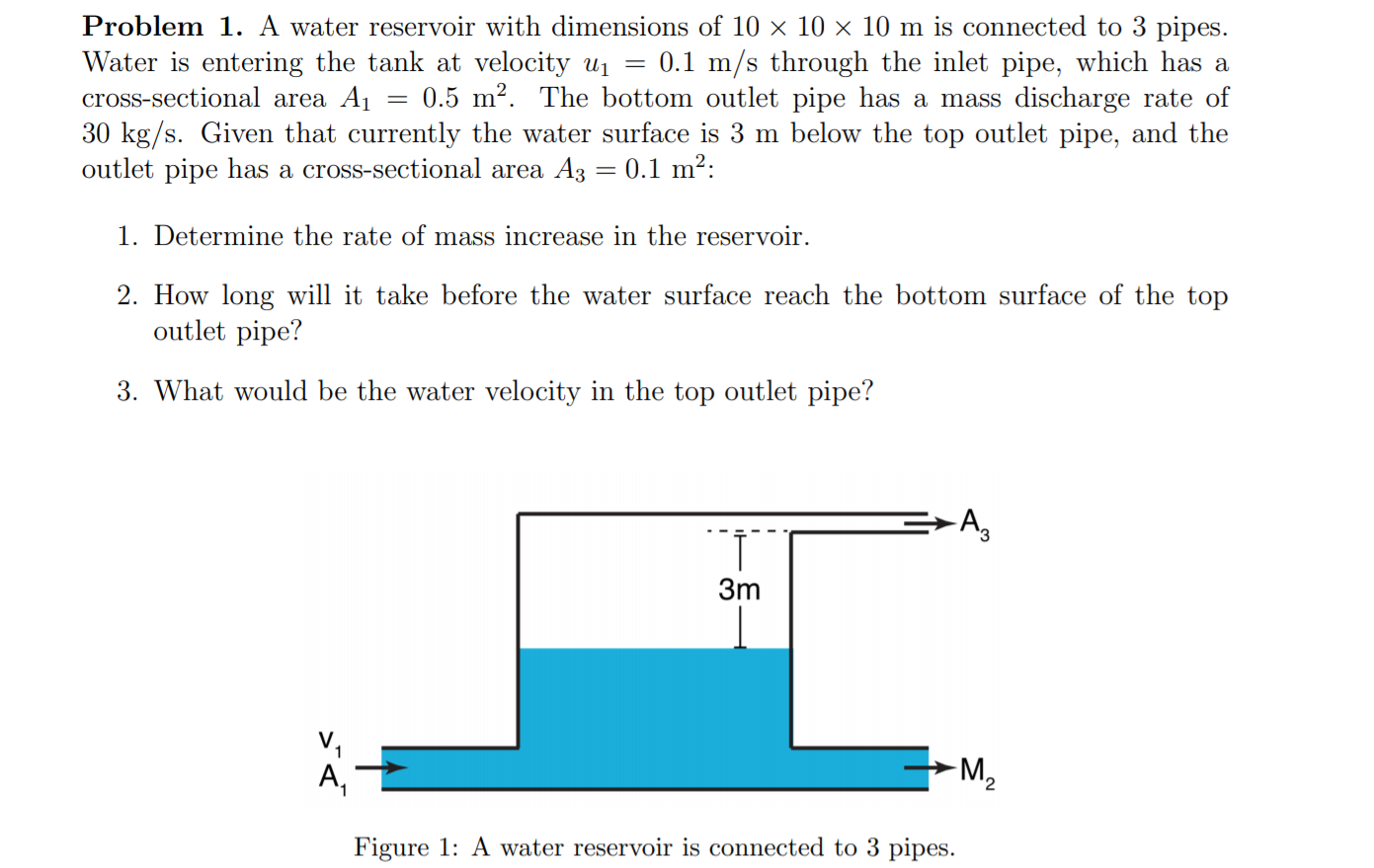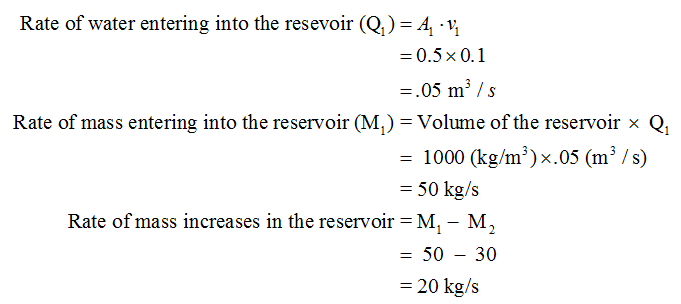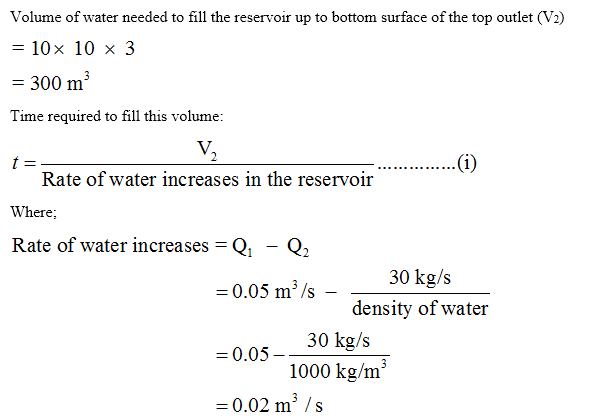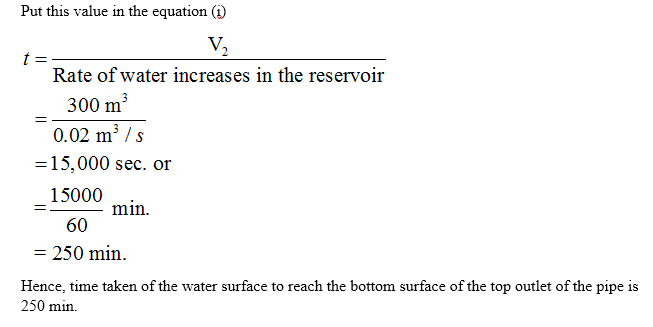# Problem 1. A water reservoir with dimensions of 10 x 10 × 10 m is connected to 3 pipes.Water is entering the tank at velocity u1 = 0.1 m/s through the inlet pipe, which has across-sectional area A10.5 m?. The bottom outlet pipe has a mass discharge rate of30 kg/s. Given that currently the water surface is 3 m below the top outlet pipe, and theoutlet pipe has a cross-sectional area A30.1 m²:1. Determine the rate of mass increase in the reservoir.2. How long will it take before the water surface reach the bottom surface of the topoutlet pipe?3. What would be the water velocity in the top outlet pipe?-A,3mV,A,-M2Figure 1: A water reservoir is connected to 3 pipes.

Question
1 viewshelp_outlineImage TranscriptioncloseProblem 1. A water reservoir with dimensions of 10 x 10 × 10 m is connected to 3 pipes. Water is entering the tank at velocity u1 = 0.1 m/s through the inlet pipe, which has a cross-sectional area A1 0.5 m?. The bottom outlet pipe has a mass discharge rate of 30 kg/s. Given that currently the water surface is 3 m below the top outlet pipe, and the outlet pipe has a cross-sectional area A3 0.1 m²: 1. Determine the rate of mass increase in the reservoir. 2. How long will it take before the water surface reach the bottom surface of the top outlet pipe? 3. What would be the water velocity in the top outlet pipe? -A, 3m V, A, -M2 Figure 1: A water reservoir is connected to 3 pipes. fullscreen
check_circle

Step 1

Given data:

Dimension of the reservoir =(10 x 10 x 10)m

water density = 1000 kg/m3     (Assumed)

v1 = 0.1 m/s

A1 = 0.5 m2

M2 = 30 kg/s

A3 = 0.1 m2

Step 2

Calculating the rate of mass increases in the reservoir:Step 3

Calculating the time taken of the water surface to reach the bottom surface of the top outlet of the pipe (t):...

### Want to see the full answer?

See Solution

#### Want to see this answer and more?

Solutions are written by subject experts who are available 24/7. Questions are typically answered within 1 hour.*

See Solution
*Response times may vary by subject and question.
Tagged in